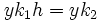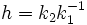# Coset containment implies subgroup containment

## Contents

DIRECT: The fact or result stated in this article has a trivial/direct/straightforward proof provided we use the correct definitions of the terms involved
View other results with direct proofs
VIEW FACTS USING THIS: directly | directly or indirectly, upto two steps | directly or indirectly, upto three steps|

## Statement

### Verbal statement

If a Left coset (?) of one subgroup is contained in a left coset of another subgroup, then the subgroup is also contained in the other.

### Statement with symbols

Suppose$xH \subseteq yK$ are cosets of subgroups$H,K \le G$. Then$H \le K$.

## Proof

Given:$xH \subseteq yK$ for some$x,y \in G$.

To prove:$H \le K$

Proof: Since$x=xe$ is in$xH$, which is contained in$yK$, there exists$k_1 \in K$ such that$x=yk_1$. Thus$yk_1H \subseteq yK$, which implies that there exist$h \in H$ and$k_2 \in K$ such that$yk_1h=yk_2$. Hence$h=k_2k_1^{-1}$, which is in$K$. So,$H\le K$.